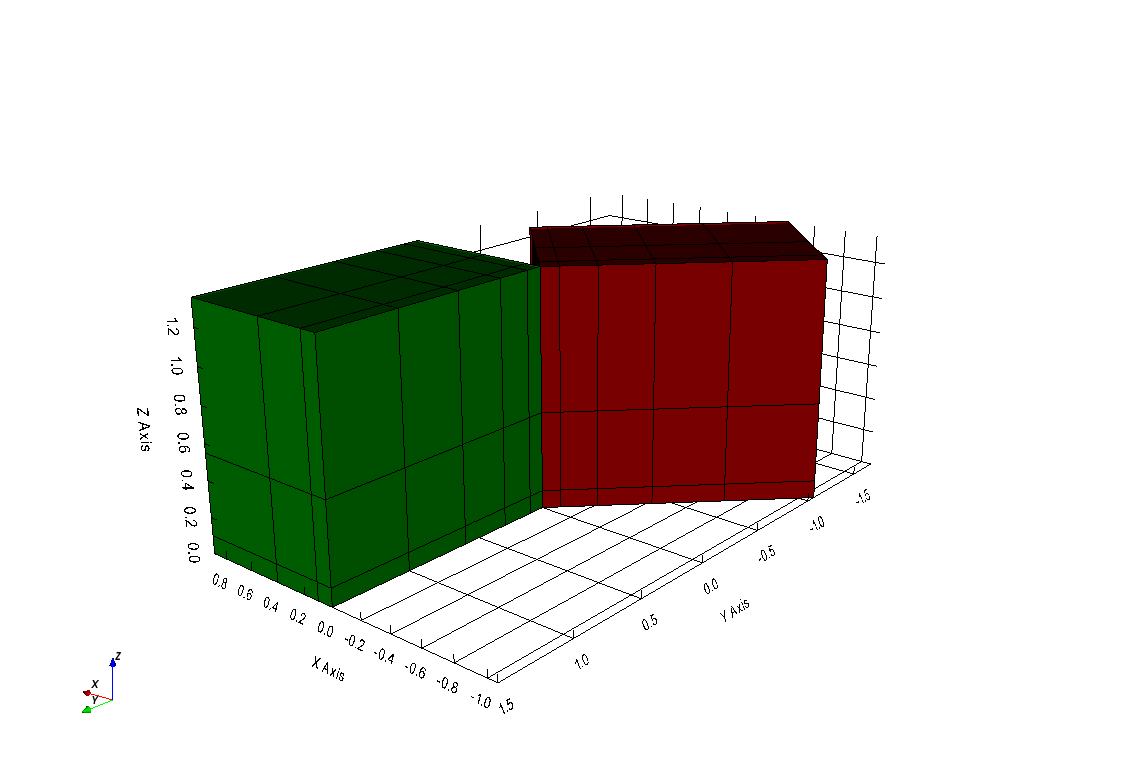# discretize.mixins.vtk_mod.InterfaceVTK¶

class discretize.mixins.vtk_mod.InterfaceVTK[source]

Bases: object

This class is full of methods that enable discretize meshes to be converted to VTK data objects (and back when possible). This is inherritted by the discretize.BaseMesh.BaseMesh class so all these methods are available to any mesh object!

CurvilinearMesh, TreeMesh, and TensorMesh are all currently implemented. The CylindricalMesh is not implemeted and will raise and excpetion. The following is an example of how to use the VTK interface to construct VTK data objects or write VTK files.

import discretize
import numpy as np
h1 = np.linspace(.1, .5, 3)
h2 = np.linspace(.1, .5, 5)
h3 = np.linspace(.1, .8, 3)
mesh = discretize.TensorMesh([h1, h2, h3])

# Get a VTK data object
dataset = mesh.to_vtk()

# Save this mesh to a VTK file
mesh.writeVTK('sample_mesh')


Note that if your mesh is defined on a reference frame that is not the traditional <X,Y,Z> system with vectors of $$(1,0,0)$$, $$(0,1,0)$$, and $$(0,0,1)$$, then the mesh will be rotated to be on the traditional reference frame. The previous example snippet provides a pyvista.RectilinearGrid object because that tensor mesh lies on the traditional reference frame. If we alter the reference frame, then we yield a pyvista.StructuredGrid that is the same mesh rotated in space.

# Defined a rotated reference frame
axis_u = (1,-1,0)
axis_v = (-1,-1,0)
axis_w = (0,0,1)
mesh.orientation = np.array([
axis_u,
axis_v,
axis_w
])

# Yield the rotated vtkStructuredGrid
dataset_r = mesh.to_vtk()

# or write it out to a VTK format
mesh.writeVTK('sample_rotated')


The two above code snippets produce a pyvista.RectilinearGrid and a pyvista.StructuredGrid respecitvely. To demonstarte the difference, we have plotted the two datasets next to eachother where the first mesh is in green and its data axes are parrallel to the traditional cartesian reference frame. The second, rotated mesh is shown in red and its data axii are rotated from the traditional cartesian refence frame as specified by the axis_u, axis_v, and axis_w properties.

import pyvista
pyvista.set_plot_theme('document')

p = pyvista.BackgroundPlotter()
p.show_grid()
p.screenshot('vtk-rotated-example.png')Methods

 toVTK([models]) Convert this mesh object to it’s proper VTK or pyvista data object with the given model dictionary as the cell data of that dataset. to_vtk([models]) Convert this mesh object to it’s proper VTK or pyvista data object with the given model dictionary as the cell data of that dataset. writeVTK(file_name[, models, directory]) Makes and saves a VTK object from this mesh and given models write_vtk(file_name[, models, directory]) Makes and saves a VTK object from this mesh and given models

## Methods¶

InterfaceVTK.toVTK(models=None)[source]

Convert this mesh object to it’s proper VTK or pyvista data object with the given model dictionary as the cell data of that dataset.

Parameters
modelsdict(numpy.ndarray)

Name(‘s) and array(‘s). Match number of cells

InterfaceVTK.to_vtk(models=None)[source]

Convert this mesh object to it’s proper VTK or pyvista data object with the given model dictionary as the cell data of that dataset.

Parameters
modelsdict(numpy.ndarray)

Name(‘s) and array(‘s). Match number of cells

InterfaceVTK.writeVTK(file_name, models=None, directory='')[source]

Makes and saves a VTK object from this mesh and given models

Parameters
file_namestr

path to the output vtk file or just its name if directory is specified

modelsdict

dictionary of numpy.array - Name(‘s) and array(‘s). Match number of cells

directorystr

directory where the UBC GIF file lives

InterfaceVTK.write_vtk(file_name, models=None, directory='')[source]

Makes and saves a VTK object from this mesh and given models

Parameters
file_namestr

path to the output vtk file or just its name if directory is specified

modelsdict

dictionary of numpy.array - Name(‘s) and array(‘s). Match number of cells

directorystr

directory where the UBC GIF file lives